### Bisected Angles

Illustration to show that the bisectors of two supplementary adjacent angles are perpendicular to each…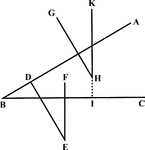### Angles With Parallel Sides

Illustration of angles with parallel sides. "GH is parallel to and lies in the same direction as DE,…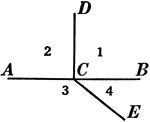### Relationships Between 4 Angles

Illustration showing four angles that can be used to define different relationships, such as adjacent,…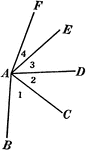### Relationships Between 4 Angles

Illustration showing four angles that can be used to define different relationships, such as adjacent,…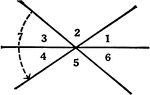### Relationships Between 6 Angles

Illustration showing six angles that can be used to define different relationships, such as adjacent,…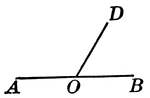### Supplementary Angles

Illustration of supplementary angles. Two angles whose sum is a straight angle.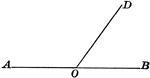### Supplementary Angles

Illustration of supplementary angles. Two angles whose sum is a straight angle. This can be used tow…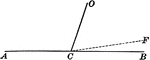### Supplementary Angles

Illustration of supplementary angles. Two angles whose sum is a straight angle. This can be used tow…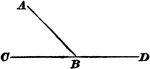### Supplementary Angles

Illustration that can be used to show supplementary angles. The angles form a straight line.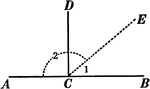### Supplementary and Right Angles

Illustration showing angles 1 and 2 are supplementary and angles ACD and DCB are supplementary. Also,…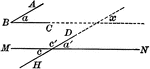### Angles With Parallel Sides

Illustration of two angles whose sides are parallel, each to each, to show they are either equal or…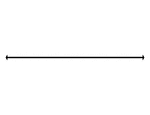### Flashcard of a Straight angle

A flashcard featuring an illustration of a Straight angle### Parallel Lines Cut By A Transversal

Illustration showing that when two parallel lines are cut by a transversal, the two interior angles…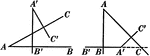### Angles With Perpendicular Sides Are Equal or Supplementary Proof

Illustration used to prove "Two angles whose sides are perpendicular each to each are either equal or…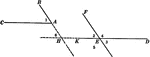### 2 Angles With Parallel Sides Theorem

Illustration used to prove the theorem, "Two angles whose sides are parallel, each to each, are either…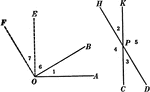### 2 Angles With Perpendicular Sides Theorem

Illustration used to prove the theorem, "Two angles whose sides are perpendicular, each to each, are…